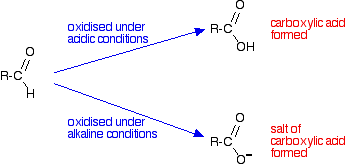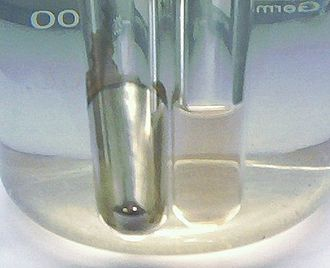# Oxidation of Aldehydes and Ketones

$$\newcommand{\vecs}{\overset { \rightharpoonup} {\mathbf{#1}} }$$ $$\newcommand{\vecd}{\overset{-\!-\!\rightharpoonup}{\vphantom{a}\smash {#1}}}$$$$\newcommand{\id}{\mathrm{id}}$$ $$\newcommand{\Span}{\mathrm{span}}$$ $$\newcommand{\kernel}{\mathrm{null}\,}$$ $$\newcommand{\range}{\mathrm{range}\,}$$ $$\newcommand{\RealPart}{\mathrm{Re}}$$ $$\newcommand{\ImaginaryPart}{\mathrm{Im}}$$ $$\newcommand{\Argument}{\mathrm{Arg}}$$ $$\newcommand{\norm}{\| #1 \|}$$ $$\newcommand{\inner}{\langle #1, #2 \rangle}$$ $$\newcommand{\Span}{\mathrm{span}}$$ $$\newcommand{\id}{\mathrm{id}}$$ $$\newcommand{\Span}{\mathrm{span}}$$ $$\newcommand{\kernel}{\mathrm{null}\,}$$ $$\newcommand{\range}{\mathrm{range}\,}$$ $$\newcommand{\RealPart}{\mathrm{Re}}$$ $$\newcommand{\ImaginaryPart}{\mathrm{Im}}$$ $$\newcommand{\Argument}{\mathrm{Arg}}$$ $$\newcommand{\norm}{\| #1 \|}$$ $$\newcommand{\inner}{\langle #1, #2 \rangle}$$ $$\newcommand{\Span}{\mathrm{span}}$$$$\newcommand{\AA}{\unicode[.8,0]{x212B}}$$

This page looks at ways of distinguishing between aldehydes and ketones using oxidizing agents such as acidified potassium dichromate(VI) solution, Tollens' reagent, Fehling's solution and Benedict's solution.

## Why do aldehydes and ketones behave differently?

You will remember that the difference between an aldehyde and a ketone is the presence of a hydrogen atom attached to the carbon-oxygen double bond in the aldehyde. Ketones don't have that hydrogen.The presence of that hydrogen atom makes aldehydes very easy to oxidize (i.e., they are strong reducing agents). Because ketones do not have that particular hydrogen atom, they are resistant to oxidation, and only very strong oxidizing agents like potassium manganate (VII) solution (potassium permanganate solution) oxidize ketones. However, they do it in a destructive way, breaking carbon-carbon bonds. Provided you avoid using these powerful oxidizing agents, you can easily tell the difference between an aldehyde and a ketone. Aldehydes are easily oxidized by all sorts of different oxidizing agents: ketones are not.

## What is formed when aldehydes are oxidized?

It depends on whether the reaction is done under acidic or alkaline conditions. Under acidic conditions, the aldehyde is oxidized to a carboxylic acid. Under alkaline conditions, this couldn't form because it would react with the alkali. A salt is formed instead.### Building equations for the oxidation reactions

If you need to work out the equations for these reactions, the only reliable way of building them is to use electron-half-equations. The half-equation for the oxidation of the aldehyde obviously varies depending on whether you are doing the reaction under acidic or alkaline conditions.

Under acidic conditions:

$RCHO + H_2O \rightarrow RCOOH + 2H^+ +2e^- \tag{1}$

Under alkaline conditions:

$RCHO + 3OH^- \rightarrow RCOO^- + 2H_2O +2e^- \tag{2}$

These half-equations are then combined with the half-equations from whatever oxidizing agent you are using. Examples are given in detail below.

## Specific examples

In each of the following examples, we are assuming that you know that you have either an aldehyde or a ketone. There are lots of other things which could also give positive results. Assuming that you know it has to be one or the other, in each case, a ketone does nothing. Only an aldehyde gives a positive result.

### Using acidified potassium dichromate(VI) solution

A small amount of potassium dichromate(VI) solution is acidified with dilute sulphuric acid and a few drops of the aldehyde or ketone are added. If nothing happens in the cold, the mixture is warmed gently for a couple of minutes - for example, in a beaker of hot water.

 ketone No change in the orange solution. aldehyde Orange solution turns green.

The orange dichromate(VI) ions have been reduced to green chromium(III) ions by the aldehyde. In turn the aldehyde is oxidized to the corresponding carboxylic acid. The electron-half-equation for the reduction of dichromate(VI) ions is:

$Cr_2O_7^{2-} + 14H^+ + 6e^- \rightarrow 2Cr^{3+} + 7H_2O \tag{3}$

Combining that with the half-equation for the oxidation of an aldehyde under acidic conditions:

$RCHO + H_2O \rightarrow RCOOH + 2H^+ +2e^- \tag{4}$

. . . gives the overall equation:

$2RCHO + Cr_2O_7^{2-} + 8H^+ \rightarrow 3RCOOH +2Cr^{3+}+ 4H_2O \tag{5}$

## Using Tollens' reagent (the silver mirror test)

Tollens' reagent contains the diamminesilver(I) ion, [Ag(NH3)2]+. This is made from silver(I) nitrate solution. You add a drop of sodium hydroxide solution to give a precipitate of silver(I) oxide, and then add just enough dilute ammonia solution to redissolve the precipitate. To carry out the test, you add a few drops of the aldehyde or ketone to the freshly prepared reagent, and warm gently in a hot water bath for a few minutes.

 ketone No change in the colorless solution. aldehyde The colorless solution produces a grey precipitate of silver, or a silver mirror on the test tube.Figure 1: Tollens' test for aldehyde: left side positive (silver mirror), right side negative. from Wikipedia

Aldehydes reduce the diamminesilver(I) ion to metallic silver. Because the solution is alkaline, the aldehyde itself is oxidized to a salt of the corresponding carboxylic acid. The electron-half-equation for the reduction of of the diamminesilver(I) ions to silver is:

$Ag(NH_3)_2^+ + e^- \rightarrow Ag + 2NH_3 \tag{6}$

Combining that with the half-equation for the oxidation of an aldehyde under alkaline conditions:

$RCHO + 3OH^- \rightarrow RCOO^- + 2H_2O +2e^- \tag{7}$

gives the overall equation:

$2Ag(NH_3)_2^+ + RCHO + 3OH^- \rightarrow 2Ag + RCOO^- + 4NH_3 +2H_2O \tag{8}$

### Using Fehling's solution or Benedict's solution

Fehling's solution and Benedict's solution are variants of essentially the same thing. Both contain complexed copper(II) ions in an alkaline solution.

• Fehling's solution contains copper(II) ions complexed with tartrate ions in sodium hydroxide solution. Complexing the copper(II) ions with tartrate ions prevents precipitation of copper(II) hydroxide.
• Benedict's solution contains copper(II) ions complexed with citrate ions in sodium carbonate solution. Again, complexing the copper(II) ions prevents the formation of a precipitate - this time of copper(II) carbonate.

Both solutions are used in the same way. A few drops of the aldehyde or ketone are added to the reagent, and the mixture is warmed gently in a hot water bath for a few minutes.

 ketone No change in the blue solution. aldehyde The blue solution produces a dark red precipitate of copper(I) oxide.Figure 2: Fehling's test. Left side negative, right side positive. from Wikipedia

Aldehydes reduce the complexed copper(II) ion to copper(I) oxide. Because the solution is alkaline, the aldehyde itself is oxidized to a salt of the corresponding carboxylic acid. The equations for these reactions are always simplified to avoid having to write in the formulae for the tartrate or citrate ions in the copper complexes. The electron-half-equations for both Fehling's solution and Benedict's solution can be written as:

$2Cu^{2+}_{complexed} + 2OH^- + 2e^- \rightarrow Cu_2O + H_2O \tag{9}$

Combining that with the half-equation for the oxidation of an aldehyde under alkaline conditions:

$RCHO + 3OH^- \rightarrow RCOO^- + 2H_2O +2e^- \tag{10}$

to give the overall equation:

$RCHO + 2Cu^{2+}_{complexed} + 5OH^- \rightarrow RCOO^- + Cu_2O + 3H_2O \tag{11}$

## Contributors

Jim Clark (Chemguide.co.uk)

This page titled Oxidation of Aldehydes and Ketones is shared under a CC BY-NC 4.0 license and was authored, remixed, and/or curated by Jim Clark.